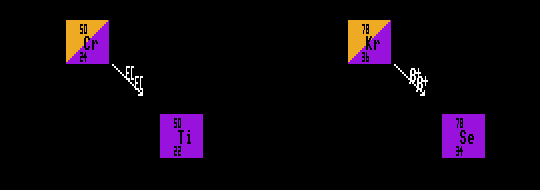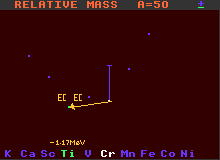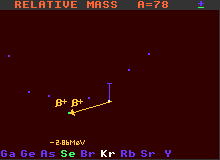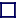### DOUBLE INVERSE BETA DECAY

DOUBLE INVERSE BETA DECAY / DOUBLE ELECTRON CAPTURE
Just as with Double Beta Decay, some nuclides with proton excesses, although unstable, are forbidden to decay by single inverse Beta decay because the immediate product has greater mass and must instead decay by double inverse Beta decay, where both occur simultaneously. This event is doubly improbable, hence such nuclides have very long half-lives. Double inverse Beta decay can only occur in nuclei that contain EVEN numbers of both protons and neutrons. Some of these so called 'even-even' nuclei cannot decay by normal inverse Beta decay because the resulting nucleus would be heavier than the original nucleus, and is thus forbidden by conservation of energy. But by changing TWO protons simultaneously into neutrons, these even-even nuclei can surmount the intervening energy barrier and reach nuclei that are lighter, so this double inverse beta decay should be allowed.A=50 Mass Diagram for the double-inverse beta-decay of Chromium-50

But since Inverse Beta decay involves the improbable action of the weak force, these double inverse beta decays are doubly improbable, and hence highly unlikely to occur often. This gives rise to nuclides with enormously long half-lives, many trillions of times longer than the present age of the Universe.A=78 Mass Diagram for the double-inverse beta-decay of Krypton-78

Examples of nuclides decaying by double inverse beta decay are chromium-50 which becomes titanium-50, and krypton-78 which becomes selenium-78. Chromium-50 has a half-life greater than 2×1017 years, and krypton-78 greater than 2×1021 years. There are about 46 nuclides that are theoretically capable of double inverse beta decay, but with the huge improbability of double inverse beta decay, not all have actually been observed to do so. To detect the decay of such nuclei requires very careful sheilding of the apparatus from background sources.

Shown is chromium-50 decaying by double Electron Capture into titanium-50, and krypton-78 decaying by double inverse beta decay into selenium-78.